Скачать презентацию ACC 412 Management Accounting I Module 4 A

• Количество слайдов: 20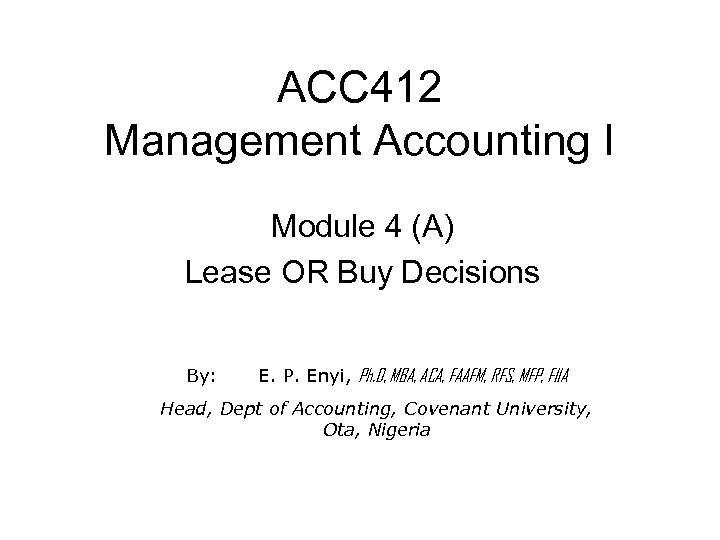ACC 412 Management Accounting I Module 4 (A) Lease OR Buy Decisions By: E. P. Enyi, Ph. D, MBA, ACA, FAAFM, RFS, MFP, FIIA Head, Dept of Accounting, Covenant University, Ota, Nigeria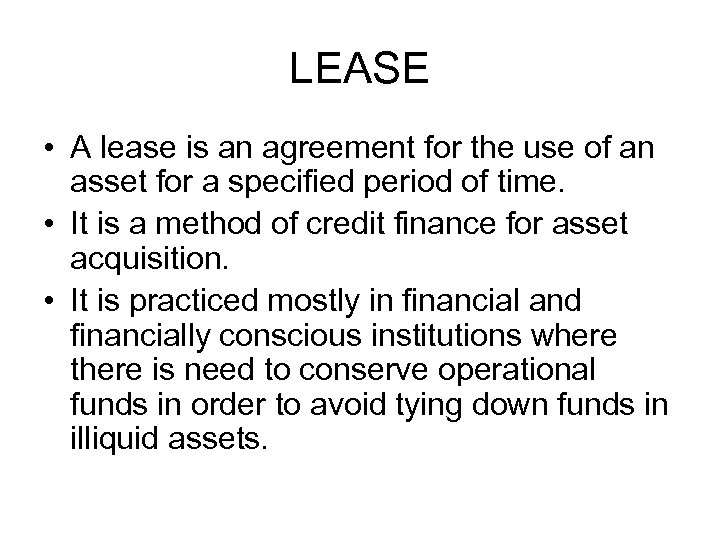LEASE • A lease is an agreement for the use of an asset for a specified period of time. • It is a method of credit finance for asset acquisition. • It is practiced mostly in financial and financially conscious institutions where there is need to conserve operational funds in order to avoid tying down funds in illiquid assets.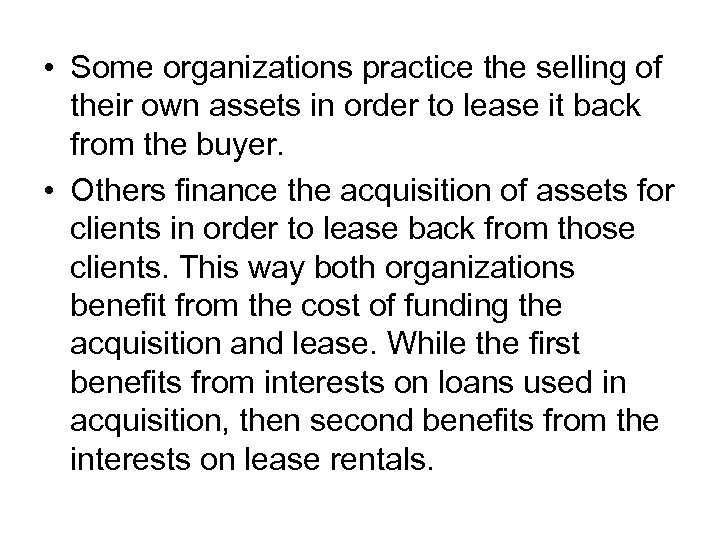• Some organizations practice the selling of their own assets in order to lease it back from the buyer. • Others finance the acquisition of assets for clients in order to lease back from those clients. This way both organizations benefit from the cost of funding the acquisition and lease. While the first benefits from interests on loans used in acquisition, then second benefits from the interests on lease rentals.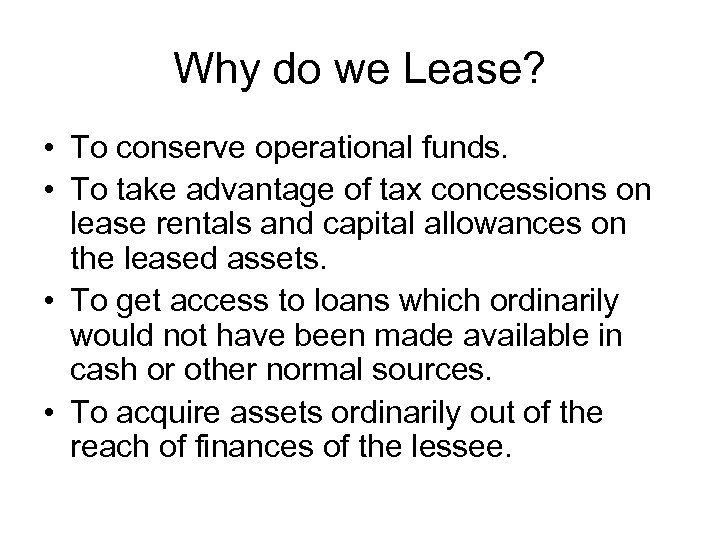Why do we Lease? • To conserve operational funds. • To take advantage of tax concessions on lease rentals and capital allowances on the leased assets. • To get access to loans which ordinarily would not have been made available in cash or other normal sources. • To acquire assets ordinarily out of the reach of finances of the lessee.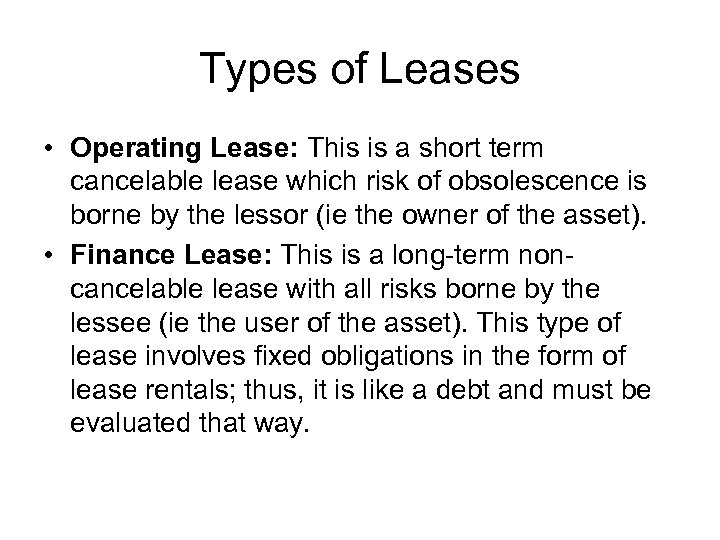Types of Leases • Operating Lease: This is a short term cancelable lease which risk of obsolescence is borne by the lessor (ie the owner of the asset). • Finance Lease: This is a long-term noncancelable lease with all risks borne by the lessee (ie the user of the asset). This type of lease involves fixed obligations in the form of lease rentals; thus, it is like a debt and must be evaluated that way.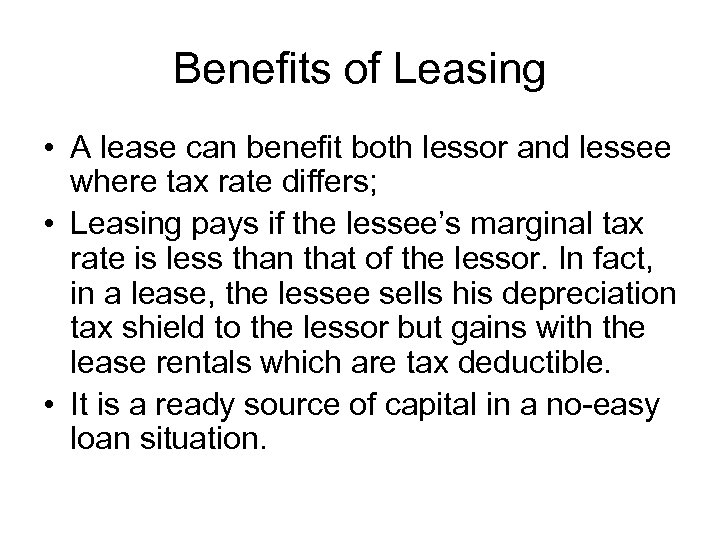Benefits of Leasing • A lease can benefit both lessor and lessee where tax rate differs; • Leasing pays if the lessee’s marginal tax rate is less than that of the lessor. In fact, in a lease, the lessee sells his depreciation tax shield to the lessor but gains with the lease rentals which are tax deductible. • It is a ready source of capital in a no-easy loan situation.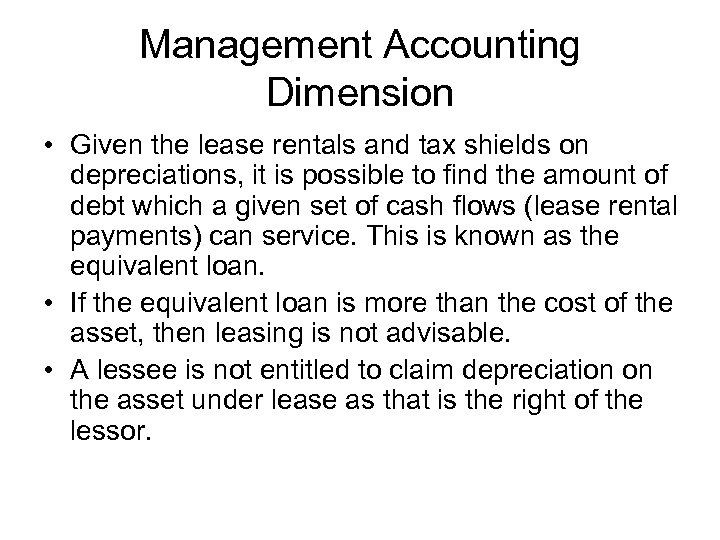Management Accounting Dimension • Given the lease rentals and tax shields on depreciations, it is possible to find the amount of debt which a given set of cash flows (lease rental payments) can service. This is known as the equivalent loan. • If the equivalent loan is more than the cost of the asset, then leasing is not advisable. • A lessee is not entitled to claim depreciation on the asset under lease as that is the right of the lessor.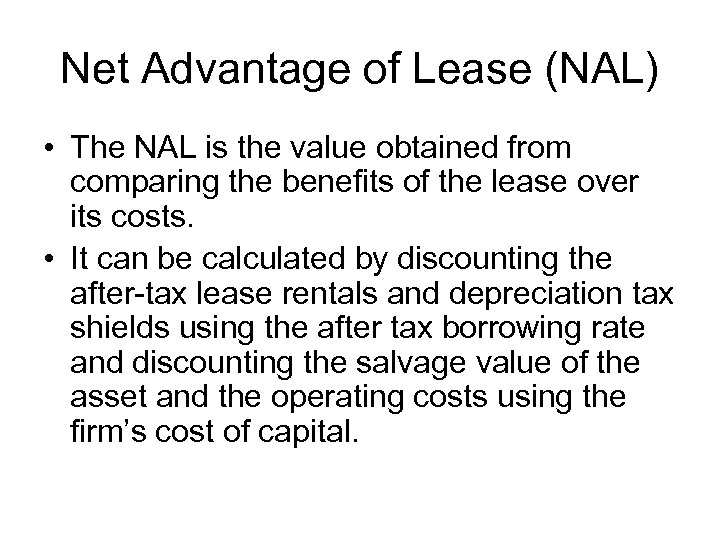Net Advantage of Lease (NAL) • The NAL is the value obtained from comparing the benefits of the lease over its costs. • It can be calculated by discounting the after-tax lease rentals and depreciation tax shields using the after tax borrowing rate and discounting the salvage value of the asset and the operating costs using the firm’s cost of capital.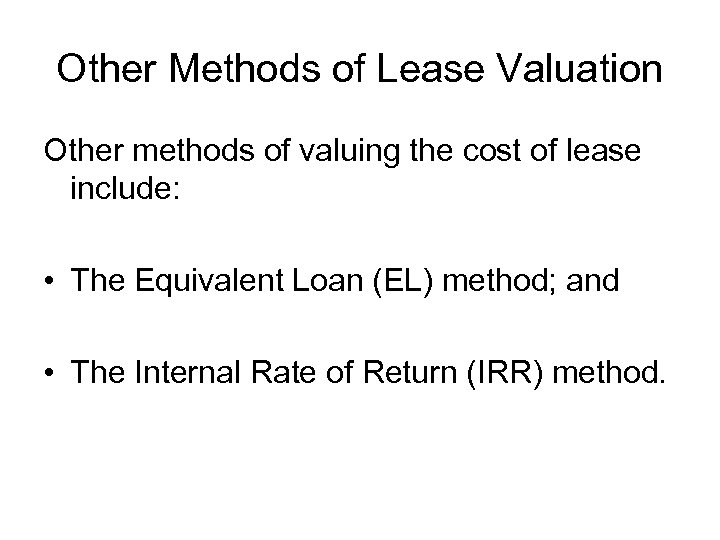Other Methods of Lease Valuation Other methods of valuing the cost of lease include: • The Equivalent Loan (EL) method; and • The Internal Rate of Return (IRR) method.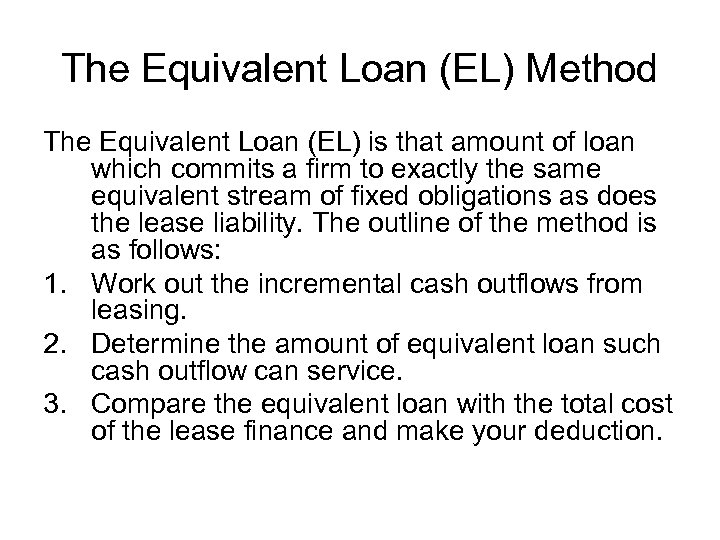The Equivalent Loan (EL) Method The Equivalent Loan (EL) is that amount of loan which commits a firm to exactly the same equivalent stream of fixed obligations as does the lease liability. The outline of the method is as follows: 1. Work out the incremental cash outflows from leasing. 2. Determine the amount of equivalent loan such cash outflow can service. 3. Compare the equivalent loan with the total cost of the lease finance and make your deduction.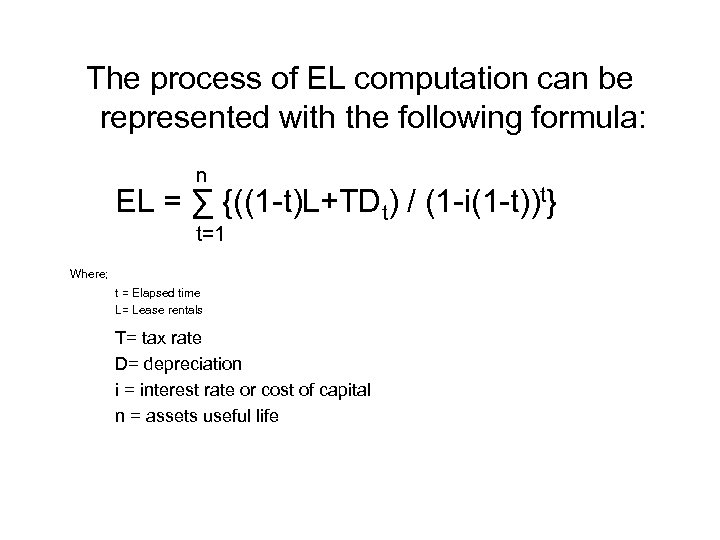The process of EL computation can be represented with the following formula: n EL = ∑ {((1 -t)L+TDt) / (1 -i(1 -t))t} t=1 Where; t = Elapsed time L= Lease rentals T= tax rate D= depreciation i = interest rate or cost of capital n = assets useful life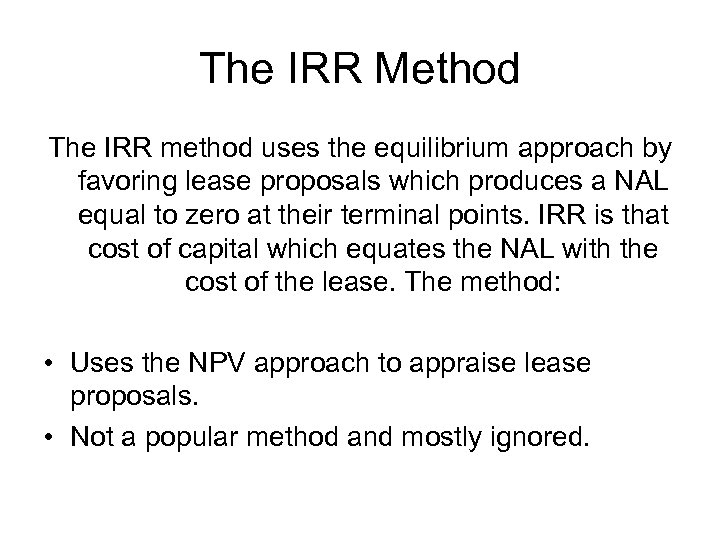The IRR Method The IRR method uses the equilibrium approach by favoring lease proposals which produces a NAL equal to zero at their terminal points. IRR is that cost of capital which equates the NAL with the cost of the lease. The method: • Uses the NPV approach to appraise lease proposals. • Not a popular method and mostly ignored.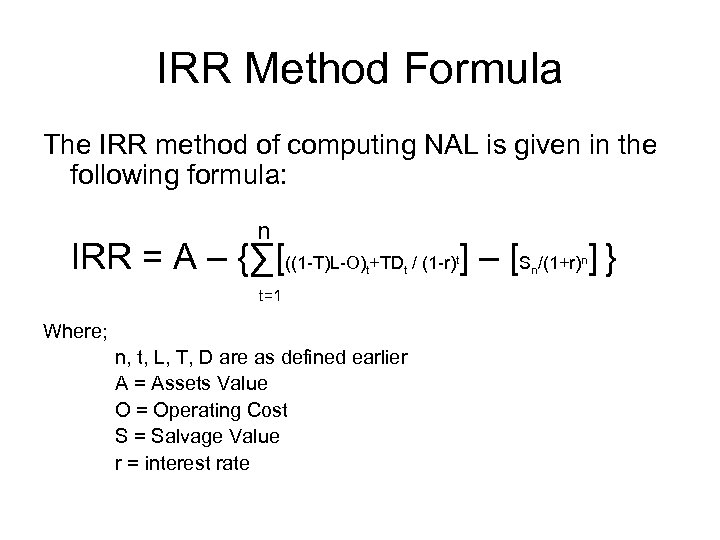IRR Method Formula The IRR method of computing NAL is given in the following formula: n IRR = A – {∑[((1 -T)L-O) +TD / (1 -r) ] – [S /(1+r) ] } t t t=1 Where; n, t, L, T, D are as defined earlier A = Assets Value O = Operating Cost S = Salvage Value r = interest rate t n n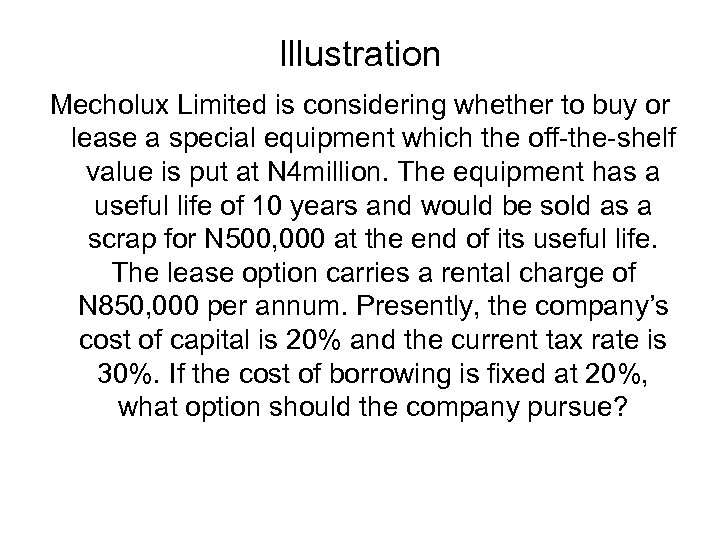Illustration Mecholux Limited is considering whether to buy or lease a special equipment which the off-the-shelf value is put at N 4 million. The equipment has a useful life of 10 years and would be sold as a scrap for N 500, 000 at the end of its useful life. The lease option carries a rental charge of N 850, 000 per annum. Presently, the company’s cost of capital is 20% and the current tax rate is 30%. If the cost of borrowing is fixed at 20%, what option should the company pursue?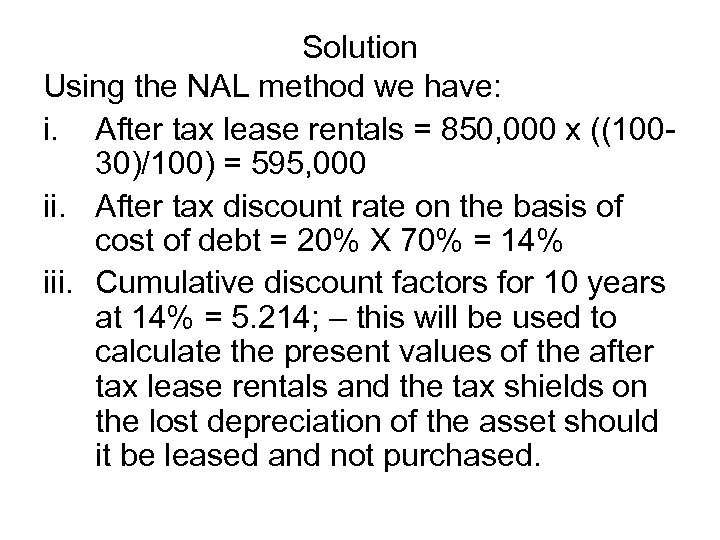Solution Using the NAL method we have: i. After tax lease rentals = 850, 000 x ((10030)/100) = 595, 000 ii. After tax discount rate on the basis of cost of debt = 20% X 70% = 14% iii. Cumulative discount factors for 10 years at 14% = 5. 214; – this will be used to calculate the present values of the after tax lease rentals and the tax shields on the lost depreciation of the asset should it be leased and not purchased.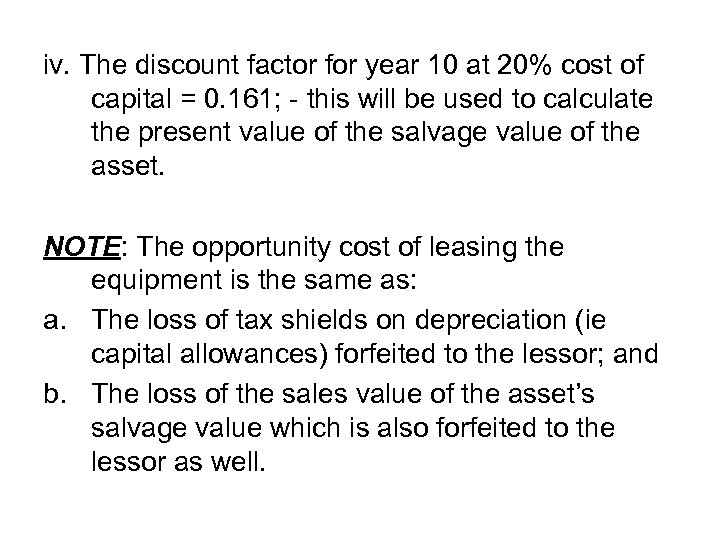iv. The discount factor for year 10 at 20% cost of capital = 0. 161; - this will be used to calculate the present value of the salvage value of the asset. NOTE: The opportunity cost of leasing the equipment is the same as: a. The loss of tax shields on depreciation (ie capital allowances) forfeited to the lessor; and b. The loss of the sales value of the asset’s salvage value which is also forfeited to the lessor as well.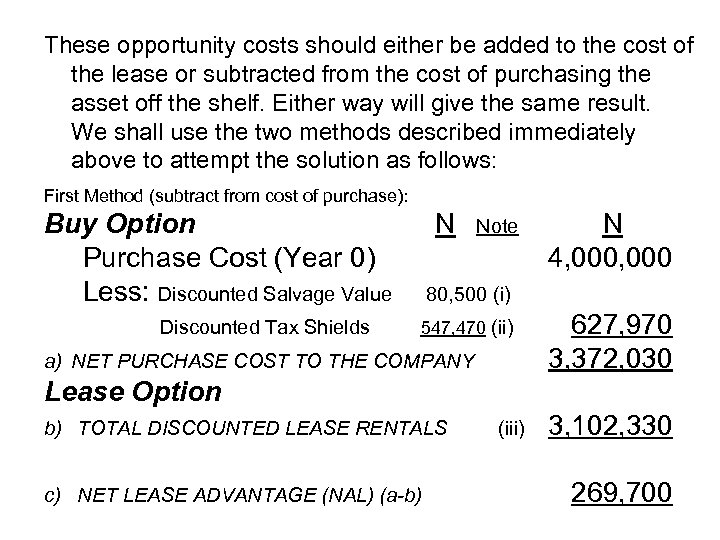These opportunity costs should either be added to the cost of the lease or subtracted from the cost of purchasing the asset off the shelf. Either way will give the same result. We shall use the two methods described immediately above to attempt the solution as follows: First Method (subtract from cost of purchase): Buy Option Purchase Cost (Year 0) Less: Discounted Salvage Value Discounted Tax Shields N Note N 4, 000 80, 500 (i) 547, 470 (ii) a) NET PURCHASE COST TO THE COMPANY 627, 970 3, 372, 030 Lease Option b) TOTAL DISCOUNTED LEASE RENTALS c) NET LEASE ADVANTAGE (NAL) (a-b) (iii) 3, 102, 330 269, 700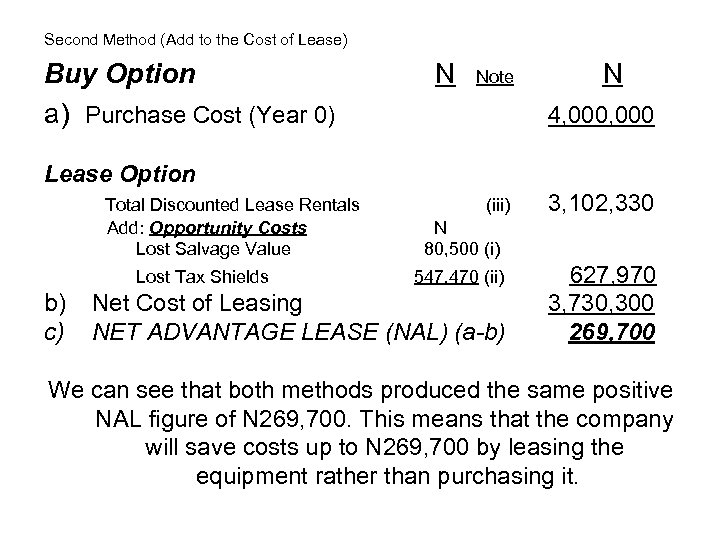Second Method (Add to the Cost of Lease) Buy Option a) Purchase Cost (Year 0) N Note N 4, 000 Lease Option Total Discounted Lease Rentals Add: Opportunity Costs Lost Salvage Value Lost Tax Shields b) c) (iii) 3, 102, 330 N 80, 500 (i) 547, 470 (ii) Net Cost of Leasing NET ADVANTAGE LEASE (NAL) (a-b) 627, 970 3, 730, 300 269, 700 We can see that both methods produced the same positive NAL figure of N 269, 700. This means that the company will save costs up to N 269, 700 by leasing the equipment rather than purchasing it.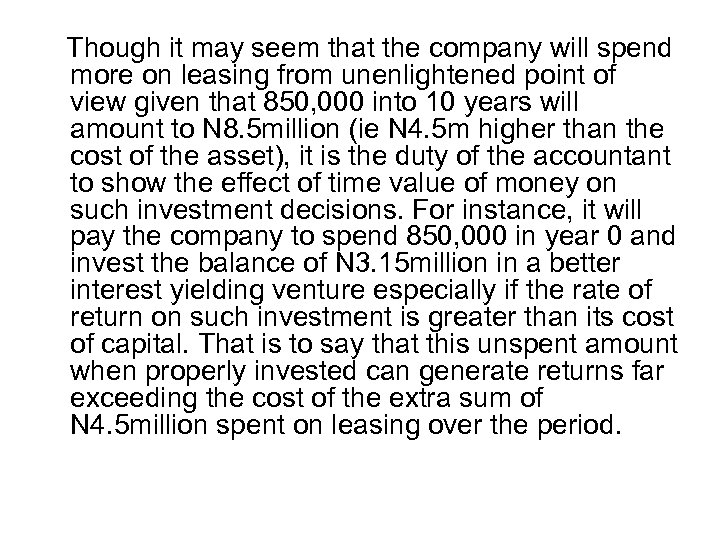Though it may seem that the company will spend more on leasing from unenlightened point of view given that 850, 000 into 10 years will amount to N 8. 5 million (ie N 4. 5 m higher than the cost of the asset), it is the duty of the accountant to show the effect of time value of money on such investment decisions. For instance, it will pay the company to spend 850, 000 in year 0 and invest the balance of N 3. 15 million in a better interest yielding venture especially if the rate of return on such investment is greater than its cost of capital. That is to say that this unspent amount when properly invested can generate returns far exceeding the cost of the extra sum of N 4. 5 million spent on leasing over the period.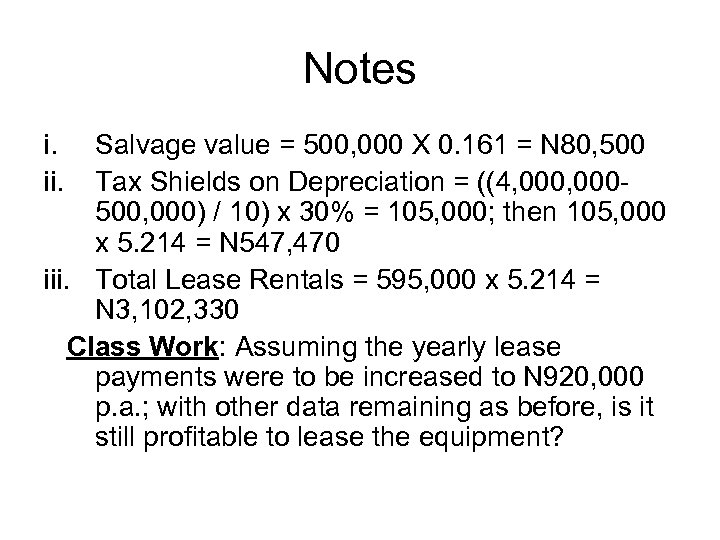Notes i. ii. Salvage value = 500, 000 X 0. 161 = N 80, 500 Tax Shields on Depreciation = ((4, 000500, 000) / 10) x 30% = 105, 000; then 105, 000 x 5. 214 = N 547, 470 iii. Total Lease Rentals = 595, 000 x 5. 214 = N 3, 102, 330 Class Work: Assuming the yearly lease payments were to be increased to N 920, 000 p. a. ; with other data remaining as before, is it still profitable to lease the equipment?